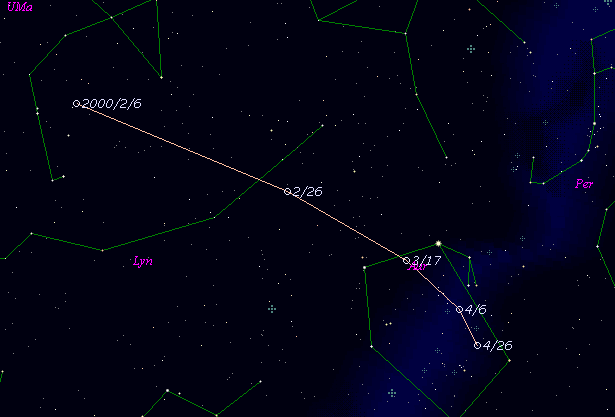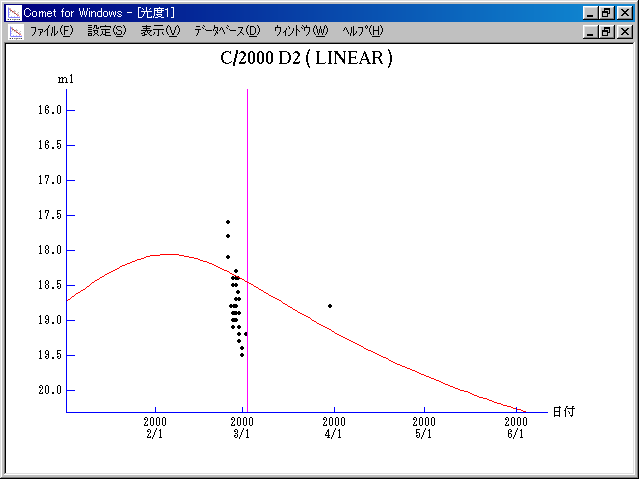# \$B%j%K%"WB@1(B

C/2000 D2 ( LINEAR )###\$B%W%m%U%#!<%k(B

 \$BH/8+F|(B 2000\$BG/(B2\$B7n(B25\$BF|(B \$BH/8+8wEY(B 18.1\$BEy(B \$BH/8+ Lincoln Laboratory Near-Earth Asteroid Research project

###\$B50F;MWAG(B

```   The following improved orbital elements by Kenji Muraoka, are
from 79 observations  2000 Feb. 25  to  Mar. 30, perturbations
by 9 Planets, Moon and 5 minor planets were taken into account.
The mean residual is +/- 0.52 arc seconds.

Epoch  =  2000 Feb. 26.0  TT       JDT = 2451600.5
T  =  2000 Mar.  8.61238       +/- 0.06348 (m.e.) TT
Peri. =  117.68216                +/- 0.02507
Node  =  235.88718                +/- 0.02940   (2000.0)
Incl. =  156.99191                +/- 0.01244
q  =    2.2976114              +/- 0.0247314 AU
e  =    0.8670410              +/- 0.0010061
a  =   17.2806047              +/- 0.1322866 AU
n  =    0.01372037             +/- 0.00015755
P  =   71.835                  +/- 0.8248721  years
(+/- 301.3 days)
```

###\$B@1?^(B###\$B8wEYJQ2=(B

```        m1 = 13.5 + 5 log\$B&\$(B + 10.0 log r
```##### \$B50F;MWAG\$OB<2,7r<#;a\$N7W;;\$K\$h\$k\$b\$N\$G\$9!#(B \$B@1?^\$O(B StellaNavigator Ver.2.0 for Windows (\$B%"%9%H%m%"!<%D(B \$BJTCx(B / \$B%"%9%-!<=PHG6I4)(B) \$B\$G:n@.\$7\$?\$b\$N\$G\$9!#(B \$B8wEY%0%i%U\$O(BComet for Windows\$B\$G:n@.\$7\$?\$b\$N\$G\$9!#(B